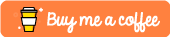# daymetpy

View the Project on GitHub khufkens/daymetpy## daymetpy: A python library for accessing Daymet surface weather data

daymetpy attempts to fill the need for easy, integrated access to gridded daily Daymet weather data. The data are hosted by the Oak Ridge National Laboratories DAAC and accessed from their web service.

## Installation

Install the package using pip and the following command:

``````pip install daymetpy
``````

## Use

Example code to calculate the temperature difference between Denver and Miami is given below. This gives an idea of code functionality and use. A worked example in an ipython notebook format can be found in the ‘example’ subdirectory.

``````import sys
import daymetpy
import pandas as pd
import seaborn as sns
import matplotlib.pyplot as plt

denver_loc = (-104.9903, 39.7392)
miami_loc = (-80.2089, 25.7753)

denver = daymetpy.daymet_timeseries(lon=denver_loc, lat=denver_loc, start_year=2012, end_year=2014)
miami = daymetpy.daymet_timeseries(lon=miami_loc, lat=miami_loc, start_year=2012, end_year=2014)

fig, ax1 = plt.subplots(1, figsize=(18, 10))
rolling3day = denver.rolling(15).mean()
ax1.fill_between(rolling3day.index, rolling3day.tmin, rolling3day.tmax,
ax1.set_title('Denver vs Miami temps (15 day mean)', fontsize=20)
rolling3day = miami.rolling(15).mean()
ax1.fill_between(rolling3day.index, rolling3day.tmin, rolling3day.tmax,
alpha=0.4, lw=0, label='Miami', color=sns.xkcd_palette(['dusty purple']))
ax1.set_ylabel(u'Temp. (°C)', fontsize=20)
fig.tight_layout()
plt.legend(fontsize=20)
``````

## Requirements

Pandas / seaborn are required.

## Contributors

• Koen Hufkens: koen.hufkens@gmail.com
• Colin Talbert